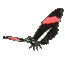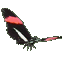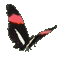Math Help - Multiply Fractions - Game Tips:

- The fraction 2/3 multiplied by 5/6 equals 10/18 , which reduces to 5/9 .
- In order to multiply two proper fractions, you multiply the numerators (tops)
and you multiply the denominators (bottoms).

- The general rule is   (a/b)(c/d) = (ac)/(bd) .

- Single denominators used in this game are less than 20. (Product denominators<300).

- Your Game Score is reduced by the number of butterfly hits.

- To slow the game speed repeat tap/click on the word Slider.
- To increase the game speed repeat tap/click on the word Math.
- Speed can also be adjusted with a keyboard's - and + keys.

- Refresh/Reload the web page to restart the game.